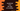# How to find the sign of a number in JavaScript## Find the sign of a number in JavaScript :

Finding the sign of a number is pretty easy in JavaScript. JavaScript provides us one inbuilt method to do that. Just call that method and it will tell you the sign. You don’t have to write any separate methods for that. In this tutorial, I will quickly show you how to do that with one example.

## Math.sign() :

sign() method is defined in Math object. You can pass one number to it and it will return the sign for that number. Following are the possible return values of this function :

``````1 => If the argument is positive
-1 => If the argument is negative
0 => If the argument is zero
-0 => If the argument is negative zero
NaN => For any other types of arguments``````

### Example to use Math.sign() :

Let me quickly show you one JavaScript example program with different types of arguments :

``````console.log(`10 : \${Math.sign(10)}`);
console.log(`-23 : \${Math.sign(-23)}`);
console.log(`0 : \${Math.sign(0)}`);
console.log(`-0 : \${Math.sign(-0)}`);
console.log(`+0 : \${Math.sign(+0)}`);
console.log(`333 : \${Math.sign(333)}`);
console.log(`NaN : \${Math.sign(NaN)}`);
console.log(`undefined : \${Math.sign(undefined)}`);
console.log(`"hello" : \${Math.sign("hello")}`);
console.log(`12.4 : \${Math.sign(12.4)}`);``````

We are using different types of arguments here. If you run this program, it will print the below output :

``````10 : 1
-23 : -1
0 : 0
-0 : 0
+0 : 0
333 : 1
NaN : NaN
undefined : NaN
"hello" : NaN
12.4 : 1``````

As you can see here, we can easily find out if a number is positive, negative or zero using this method. Try to run the above example with different parameter values and drop a comment below if you have any queries.